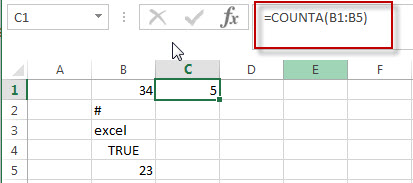# Excel COUNTA Function

This post will guide you how to use Excel COUNTA function with syntax and examples in Microsoft excel.

## Description

The Excel COUNTA function counts the number of cells that are not empty in a range. And it returns the number of non-blank cells within a range or values. It can be used to count all the cells in a range that contain numbers, text, logical values, error values and empty text string.

The COUNTA function is a build-in function in Microsoft Excel and it is categorized as a Statistical Function.

The COUNTA function is available in Excel 2016, excel 2013, excel 2010, excel 2007, excel 2003, excel XP, excel 2000, excel 2011 for Mac.

## Syntax

The syntax of the COUNTA function is as below:

`= COUNTA(value1, [value2],…)`

Where the COUNTA function arguments are:

• value1 -This is a required argument.  The first item, cell reference, or range within which you want to count numbers.
• Value2 – This is an optional argument. You can enter up to 255 value arguments.

Note:

• The COUNTA function does not count empty cells.
• If you want to only count cells that contain numbers, just use the COUNT function.
• If you want to count logical values, text, or error values, you can use the COUNTA function.
• You can provide up to 255 number arguments in excel 2007 or later. If you are using excel 2003, you can only provide up to 30 number arguments.

### Excel COUNTA Function Examples

The below examples will show you how to use Excel COUNTA Function to count the number of cells in a range that are not empty.

#1 To count the number of cells in the range B1:B4, just using the following excel formula:

=COUNTA(B1:B4)### More Excel CountA Function Examples

• Generate All Possible Combinations of Two Lists
You can use a formula based on the IF function, the ROW function, the COUNTA function, The INDEX function and the MOD function to get a list of all possible combinations from those two list….